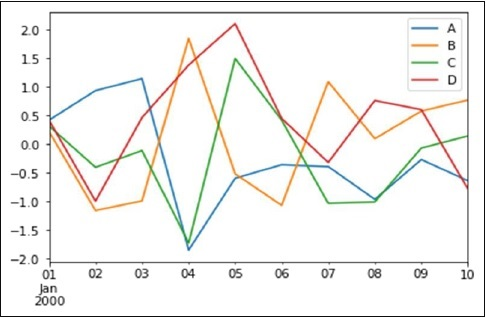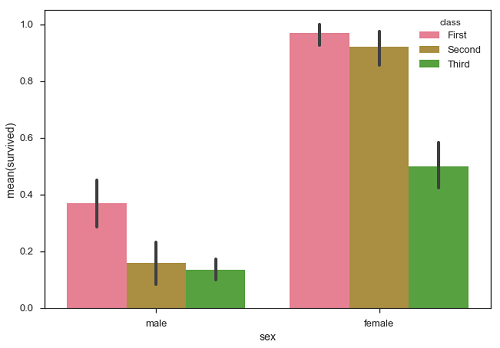# File Handling | Python

Topics Discussed: 1)Opening a File 2)Reading from a file 3)Closing a file Python for beginners: https://www.youtube.com/watch?v=egq7Z...

Telegram: https://t.me/clcoding_python

# Rules for Python variables

Rules for Python variables:
A variable name must start with a letter or the underscore character
A variable name can only contain alpha-numeric characters and underscores (A-z, 0-9, and _ )
Variable names are case-sensitive (age, Age and AGE are three different variables)

Telegram: https://t.me/clcoding_python

# Export Pandas DataFrame to CSV

Topics discussed: 1)count the number of rows in a Pandas DataFrame in Python 2)count the number of columns in a Pandas DataFrame in Python 3)Extracting specific column in Pandas DataFrame in Python 4) Filter Pandas Dataframe 5)Export Pandas DataFrame to CSV Prerequisite: Read csv using pandas.read_csv() | Python | Castor Classes https://www.youtube.com/watch?v=Sgqry... Python for beginners: https://www.youtube.com/watch?v=egq7Z...

# Uncommon Words from Two Sentences | Python

Problem Statement: We are given two sentences A and B. (A sentence is a string of space separated words. Each word consists only of lowercase letters.) A word is uncommon if it appears exactly once in one of the sentences, and does not appear in the other sentence. Return a list of all uncommon words. You may return the list in any order. Example 1: Input: A = "this apple is sweet", B = "this apple is sour" Output: ["sweet","sour"] Example 2: Input: A = "apple apple", B = "banana" Output: ["banana"] Code is given in the comment section. Prerequisite: Counting the frequencies in a list using dictionary | Python | Castor Classes https://www.youtube.com/watch?v=yZKGU... Python for beginners: https://www.youtube.com/watch?v=egq7Z...

# Counting the frequencies in a list using dictionary | Python

Code:
num1=[1,1,2,3,2,5,7,5];
dict1={};
for num in num1:
if num in dict1:
dict1[num]=dict1[num]+1;
else:
dict1[num]=1;

Python for beginners:

# Dictionary Part 4 | Python

Prerequisite: Dictionary | Python | Castor Classes https://www.youtube.com/watch?v=yZTR5... Dictionary Part 2 | Python | Castor Classes https://www.youtube.com/watch?v=qU1dV... Dictionary Part 3 | Python | Castor Classes https://www.youtube.com/watch?v=nFfxX... Python for beginners: https://www.youtube.com/watch?v=egq7Z...

# Linear Regression in python | python crash course_06

## Linear Regression in Machine learning :

Welcome to machine learning in python crash course, so in this section we will learn different machine learning algorithm. Let's start:
Linear Regressions is usually the first machine learning algorithm. It is a simple model but everyone need to master it as it lays the foundation for other machine learning algorithm.

## It is a very powerful techniques and can be used to understand the factors that influence profitability. It can be used to forecast sale in the coming months by analyzing the sales data for previous month. It can also be used to gain various insights into customers behaviour. By the end of the blog, we will build a model which looks like the below picture i.e, determine a line which best fit the data. Example In this example, we will use Pima Indian Diabetes dataset to select four of the attributes having best features with the help of chi-square statistical test. ``` from pandas import read_csv from numpy import set_printoptions from sklearn.feature_selection import SelectKBest from sklearn.feature_selection import chi2 path = r'C:\pima-indians-diabetes.csv' names = ['preg', 'plas', 'pres', 'skin', 'test', 'mass', 'age', 'class'] dataframe = read_csv(path, names=names) array = dataframe.value ```

Next, we will separate array into inputs and outputs components −
X = array[:,0:8]

Y = array[:,8]
The following line of code will select the best features from dataset −
test = SelectKBest(score_func=chi2, k=4)

fit = test.fit(X,Y)
set_printoptions(precision=2)
print(fit.scores_)
featured_data = fit.transform(X)
print ("\nFeatured data:\n", featured_data[0:4])

OUTPUT:
[ 111.52 1411.89 17.61 53.11 2175.57 127.67 5.39 181.3
Featured data:
[[148.  0. 33.6 50.
[  89. 94. 28.1 21. ]]
[  85.  0. 26.6 31. ]
[ 183.  0. 23.3 32. ]

BEST OF LUCK!!!!!
Telegram: https://t.me/clcoding_python

# Pandas Built in Data Visualization | python crash course_05

## Pandas Built in Data Visualization:

Welcome to python crash course tutorial, today we will see the last topic Pandas Built in Data Visualization in the data science section.
SOME INTRODUCTION:
Data Visualizations is the presentation of data in graphical format. It help people understand the significance of data by summarizing and presenting a huge amount of data in a simple and easy-to-understand format and help communicate information clearly and effectively.
In this tutorial, we will learn about pandas built-in capabilities for data visualizations. It is built-off of matplotlib, but it baked into pandas for easier usage.
Let’s take a looks
Example:
Basic Plotting: plot

This functionality on Series and DataFrame is just a simple wrapper around the matplotlib libraries plot() methods.
```
import pandas as pd

import numpy as np

df = pd.DataFrame(np.random.randn(10,4),index=pd.date_range('1/1/2000',

periods=10), columns=list('ABCD'))

df.plot()
```
Output:If the index consists of dates, it calls gct().autofmt_xdate() to format the x-axis as shown in the above illustration.
We can plots one column versus another using the x and y keywords.
Plotting method allow a handful of plot styles other than the default line plot. These method can be provided as the kind keyword argument to plots(). These include −
1. bar or barh for bar plots
2. hist for histogram
3. box for boxplot
4. 'area' for area plots
5. 'scatter' for scatter plots

BEST OF LUCK!!!

# Dictionary Part 3 | Python

Topics covered: 1)pop method in Dictionary in Python 2)delete statement in Dictionary in Python 3)clear method in Dictionary in Python Prerequisite: Dictionary | Python | Castor Classes https://www.youtube.com/watch?v=yZTR5... Dictionary Part 2 | Python | Castor Classes https://www.youtube.com/watch?v=qU1dV... Python for beginners: https://www.youtube.com/watch?v=egq7Z...

Telegram: https://t.me/clcoding_python

# Dictionary Part 2 | Python

Topics covered: 1)How to print Dictionary 2)How to add / append key value pairs in dictionary 3)Update a Dictionary using Assignment Prerequisite: Dictionary | Python | Castor Classes https://www.youtube.com/watch?v=yZTR5... Python for beginners: https://www.youtube.com/watch?v=egq7Z...

Telegram: https://t.me/clcoding_python

# Efficient way to compute prefix sum | Python

Prerequisite:
https://www.youtube.com/watch?v=ESVXW... Check this link for more detail explanation of this powerful algorithm: https://en.wikipedia.org/wiki/Prefix_sum Python for beginners: https://www.youtube.com/watch?v=egq7Z...

Code: l=eval(input()); i=1; output=[]; output.append(l); prefixsum=l; while(i<len(l)): prefixsum=prefixsum+l[i]; output.append(prefixsum); i=i+1; print(output)

Telegram: https://t.me/clcoding_python

# What is Seaborn in data visualization? | python crash course_04

## What is Seaborn in data visualization?

Hello everyone,  In the Previous blog we see first part of data visualization (Matplotlib) This blog is related to Seaborn. so Let's start:

## Keys Feature

1. Seaborns is a statistical plotting library
2. It has beautiful default style
3. It also is designed to work very well with Pandas dataframe object.

## Installing and getting started:

To install the latest release of seaborn, you can use:
pip install seaborn
conda install seaborn
Alternatively, you can used pip to install the development version directly from github:
Another option would be to to clone the github repository and install from yours local copy:
pip install . Python 2.7 or 3.5+

## Bar Plots

The barplots() shows the relation between a categorical variable and a continuous variable. The data is represented in rectangular bar where the length the bar represent the proportion of the data in that category.

Bar plots represents the estimate of central tendency. Let us use the ‘titanic’ dataset to learn bar plot.

### Example

```
import pandas as pd

import seaborn as sb

from matplotlib import pyplot as plt

sb.barplot(x = "sex", y = "survived", hue = "class", data = df)

plt.show()
```

### Output:BEST OF LUCK!!!!!

# Reverse a string using Stack | Python

Prerequisite: Reverse First K elements of Queue using STACK | Python | Castor Classes https://www.youtube.com/watch?v=gqIA2... Python for beginners: https://www.youtube.com/watch?v=egq7Z...

Code: def reverse(string): #Add code here stack=[]; for i in string: stack.append(i); s=""; i=0; while(i<len(string)): s=s+stack.pop(-1); i=i+1; return s;

Telegram: https://t.me/clcoding_python